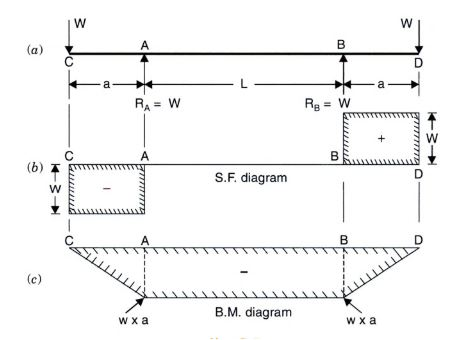# Simple Bending Theory-Bending Stress-Bending Equation-Derivation

Simple bending or pure bending is defined as the phenomenon of development of stresses throughout the length of the beam due to the action of bending moment exclusively. The stresses development throughout the length of the beam is called as bending stress.
In this article, we will discuss the simple bending theory, the assumptions in the theory followed by the derivation to determine the bending stress or the Bending equation.Beam Construction; Image Courtesy: Molin Precast Products Also Watch: What is a Flexural Member?

### Simple Bending Theory

Simple bending can be explained by the below beam and load arrangement. As shown in the figure, from the BM diagram of the corresponding beam arrangement have constant Bending moment along the beam length AB and the SF has nothing to contribute to that length.Fig.1. Simple Bending Theory Explanation
This beam section AB is said to be in the condition of simple bending or pure bending.

### Assumptions in Simple Bending Theory

The assumptions in simple bending theory are:
1. The material of the beam is homogeneous and isotropic
2. The transverse section of the beam remains plane before and after bending.
3. The value of young's modulus is the same in tension and compression
4.  The beam is initially straight and all the longitudinal filaments bend into circular arcs with a common center of curvature
5. The radius of curvature is large compared with the dimensions of the cross-section.
6. Every layer of the beam is free to expand or contract independent of the layer below it.

### Simple Bending Theory

Simple bending theory can be explained by following beam element consideration, as shown in figure-2 below.

As shown in the figure above, consider a beam small beam section ABCD with a length of dx. The N-N forms the neutral axis of the beam element. Section AB and CD are perpendicular to the neutral axis N-N.  The beam element under the action of bending gets deformed as shown in figure-2(b).

The layers of the beam before bending do not remain the same after bending. The layer AC and BD have deformed to A'C' and B'D' respectively. The layer gets shorten at top and expands at the bottom layer. The beam layer does not undergo any change at the neutral layer. This means,
NN = dx = N'N'
The layers above the neutral axis are shortened, hence subjected to a compressive force. The layer below the neutral axis is elongated, thus subjected to tensile stress. Hence, the compressive stress is maximum at the top layer and the tensile stress is maximum at the bottom layer.

As we move from the bottom layer to the neutral layer, the length of layers decreases. Hence, the increase or decrease of length of the layer is dependent on its distance from the neutral axis. This theory of bending is called the theory of simple bending.

### Derivation of Bending Equation

As shown in figure-2(a), consider a layer EF from a distance 'y' from the neutral axis. After bending, EF gets deformed to E'F' as shown in 2(b).

Given:
1. The radius of the Neutral layer = R
2. The angle subtended by A'B' and C'D' at O = θ
Then,

#### Strain Variation along Depth of Beam

Original length of layer = EF = dx;
Original length of Neutral layer = NN = N'N' = dx
From Figure,

N'N' = R x  θ = dx
E'F' = (R + y ) θ

Strain in the layer EF = Increase in the length of layer EF / (Original Length)
= (E'F' -EF)/EF
= ( (R +y) θ - (Rx θ))/dx
= (R θ + y θ - R θ)/ dx
= y θ/dx
= y θ/R θ
=y/R
Hence,
the strain in the layer EF is directly proportional to the distance of the layer from the neutral axis. This relation shows the vairation of the strain along the depth of the beam. The variation of strain is linear.

#### Stress Variation along Depth of Beam

Young's modulus E = stress in layer EF / Strain in layer EF =  𝛔 /(y/R)

=> E = 𝜎R/y

=> E/R = 𝛔/y
• Moment of Resistance
The layer above the neutral axis experience compressive force Fc and the layers below the neutral axis experiences tensile force Ft. For equilibrium:
1. Fc= Ft
2. The moments caused due to these internal forces must be equal and opposite the BM caused at section f the beam.
Moment of resistance is defined as the algebraic sum of moments about the neutral axis of the internal forces developed in the beam.

If the cross-sectional area of the element is dA, then

Total Moment of resistance M = ∫ (𝛔 dA . y) = ∫(Ey. y. dA)/R
=> M = (E/R)∫y^2 . dA

Moment of Inertia I = ∫y^2. dA

Hence, we get
M = EI/R

Hence, we get

M/I = E/R = 𝛔/y ;

The above equation is called as the Bending Equation

Watch Video on #Types of #Foundation in #Construction